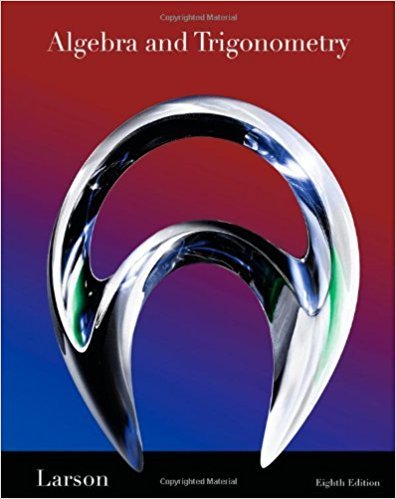×
×

# Solutions for Chapter 6.7: Applications and Models## Full solutions for Algebra and Trigonometry | 8th Edition

ISBN: 9781439048474Solutions for Chapter 6.7: Applications and Models

Solutions for Chapter 6.7
4 5 0 359 Reviews
22
5
##### ISBN: 9781439048474

This expansive textbook survival guide covers the following chapters and their solutions. Since 70 problems in chapter 6.7: Applications and Models have been answered, more than 47407 students have viewed full step-by-step solutions from this chapter. This textbook survival guide was created for the textbook: Algebra and Trigonometry, edition: 8. Chapter 6.7: Applications and Models includes 70 full step-by-step solutions. Algebra and Trigonometry was written by and is associated to the ISBN: 9781439048474.

Key Math Terms and definitions covered in this textbook
• Back substitution.

Upper triangular systems are solved in reverse order Xn to Xl.

• Circulant matrix C.

Constant diagonals wrap around as in cyclic shift S. Every circulant is Col + CIS + ... + Cn_lSn - l . Cx = convolution c * x. Eigenvectors in F.

• Commuting matrices AB = BA.

If diagonalizable, they share n eigenvectors.

• Ellipse (or ellipsoid) x T Ax = 1.

A must be positive definite; the axes of the ellipse are eigenvectors of A, with lengths 1/.JI. (For IIx II = 1 the vectors y = Ax lie on the ellipse IIA-1 yll2 = Y T(AAT)-1 Y = 1 displayed by eigshow; axis lengths ad

• Kirchhoff's Laws.

Current Law: net current (in minus out) is zero at each node. Voltage Law: Potential differences (voltage drops) add to zero around any closed loop.

• Least squares solution X.

The vector x that minimizes the error lie 112 solves AT Ax = ATb. Then e = b - Ax is orthogonal to all columns of A.

• Left inverse A+.

If A has full column rank n, then A+ = (AT A)-I AT has A+ A = In.

• Minimal polynomial of A.

The lowest degree polynomial with meA) = zero matrix. This is peA) = det(A - AI) if no eigenvalues are repeated; always meA) divides peA).

• Multiplicities AM and G M.

The algebraic multiplicity A M of A is the number of times A appears as a root of det(A - AI) = O. The geometric multiplicity GM is the number of independent eigenvectors for A (= dimension of the eigenspace).

• Normal equation AT Ax = ATb.

Gives the least squares solution to Ax = b if A has full rank n (independent columns). The equation says that (columns of A)·(b - Ax) = o.

• Outer product uv T

= column times row = rank one matrix.

• Positive definite matrix A.

Symmetric matrix with positive eigenvalues and positive pivots. Definition: x T Ax > 0 unless x = O. Then A = LDLT with diag(D» O.

• Rank one matrix A = uvT f=. O.

Column and row spaces = lines cu and cv.

• Reduced row echelon form R = rref(A).

Pivots = 1; zeros above and below pivots; the r nonzero rows of R give a basis for the row space of A.

• Similar matrices A and B.

Every B = M-I AM has the same eigenvalues as A.

• Standard basis for Rn.

Columns of n by n identity matrix (written i ,j ,k in R3).

• Trace of A

= sum of diagonal entries = sum of eigenvalues of A. Tr AB = Tr BA.

• Triangle inequality II u + v II < II u II + II v II.

For matrix norms II A + B II < II A II + II B II·

• Tridiagonal matrix T: tij = 0 if Ii - j I > 1.

T- 1 has rank 1 above and below diagonal.

• Unitary matrix UH = U T = U-I.

Orthonormal columns (complex analog of Q).

×